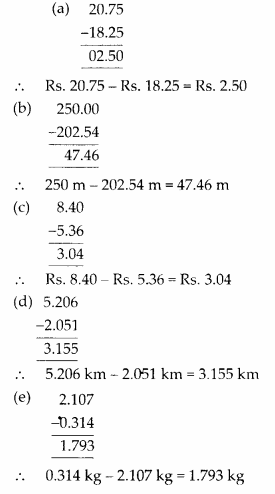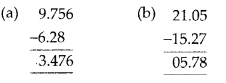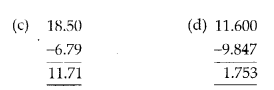# MP Board Class 6th Maths Solutions Chapter 8 Decimals Ex 8.6

## MP Board Class 6th Maths Solutions Chapter 8 Decimals Ex 8.6

Question 1.
Subtract:
(a) Rs. 18.25 from Rs. 20.75
(b) 202.54 m from 250 m
(c) Rs. 5.36 from Rs. 8.40
(d) 2.051 km from 5.206 km
(e) 0.314 kg from 2.107 kg
Solution:Question 2.
Find the value of:
(a) 9.756 – 6.28
(b) 21.05 – 15.27
(c) 18.5 – 6.79
(d) 11.6 – 9.847
Solution:Question 3.
Raju bought a book for Rs. 35.65. He gave Rs. 50 to the shopkeeper. How much money did he get back from the shopkeeper?
Solution:
Total amount given to shopkeeper = Rs. 50
Cost of book = Rs. 35.65
Amount left = Rs. 50.00 – Rs. 35.65 = Rs. 14.35
Therefore, Raju got back Rs. 14.35 from the shopkeeper.Question 4.
Rani had Rs. 18.50. She bought one ice-cream for Rs. 11.75. How much money does she have now?
Solution:
Total money = Rs. 18.50
Cost of ice-cream = Rs. 11.75
Amount left = Rs. 18.50 – Rs. 11.75 = Rs. 6.75
Therefore, Rani has Rs. 6.75 now.

Question 5.
Tina had 20 m 5 cm long cloth. She cuts 4 m 50 cm length of cloth from this for making a curtain. How much cloth is left with her?
Solution:
Total length of cloth = 20 m 5 cm = 20.05 m
Length of cloth used = 4 m 50 cm = 4.50 m
Remaining cloth = 20.05 m – 4.50 m = 15.55 m
Therefore, 15.55 m of cloth is left with Tina.

Question 6.
Namita travels 20 km 50 m every day. Out of this she travels 10 km 200 m by bus and the rest by auto. How much distance does she travel by auto?
Solution:
Total distance travelled = 20 km 50 m = 20.050 km
Distance travelled by bus = 10 km 200 m = 10.200 km
Distance travelled by auto = (20.050 – 10.200) km = 9.850 km
Therefore, 9.850 km distance is travelled by auto.Question 7.
Aakash bought vegetables weighing 10 kg. Out of this, 3 kg 500 g is onions, 2 kg 75 g is tomatoes and the rest is potatoes. What is the weight of the potatoes?
Solution:
Weight of onions = 3 kg 500 g = 3.500 kg
Weight of tomatoes = 2 kg 75 g = 2.075 kg
Total weight of onions and tomatoes
= (3.500 + 2.075) kg = 5.575 kg
Therefore, weight of potatoes
= (10.000 – 5.575) kg = 4.425 kg
Thus, weight of potatoes is 4.425 kg.